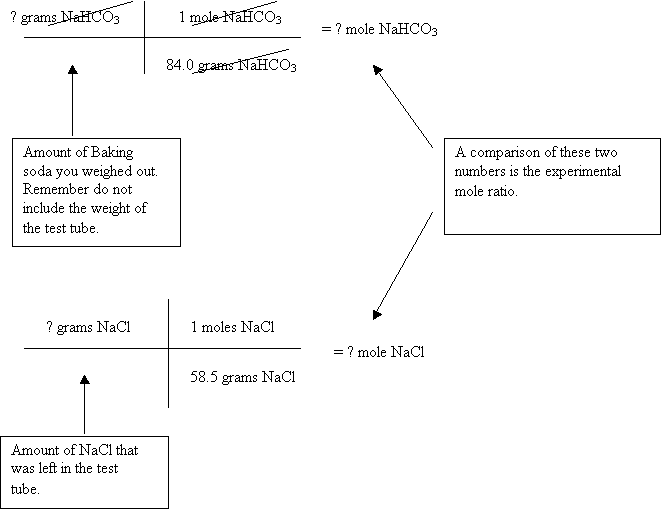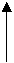If you clicked on the help link for calculating what they were asking for in this section than you should review your notes. There are many examples of mole ratio problems located in there. Now if you are one who can’t read your own handwriting or your dog ate your notes here is a little extra help.

The first thing you need to do is write the equation down of what ever your working on. In this case baking soda was mixed with hydrochloric acid. What you noticed when you mixed this was a lot of fizzing and when boiled down a powder was formed, and “NO” it is not “coke cane”. The white power is ordinary table salt, NaCl. But what is the gas produced. A little hint, it is the same stuff that is in “Pop”.

I will not place give you the equation, but I begin to set it up for you.

NaHCO3 + HCl   ®  NaCl   +   mystery gas   + H2O

Once you have the formulas correct be sure to balance the equation.

The next thing is to do a mass to mass problem starting off with the given quantity of NaHCO3.  Remember this from the notes it has three steps.The theoretical mole ratio is a comparison of the number of moles needed in the equation. These are simply the coefficients for the NaHCO3 and NaCl. If you remember coefficients are whole numbers unlike what you’re going to get from the experimental mole ratio.